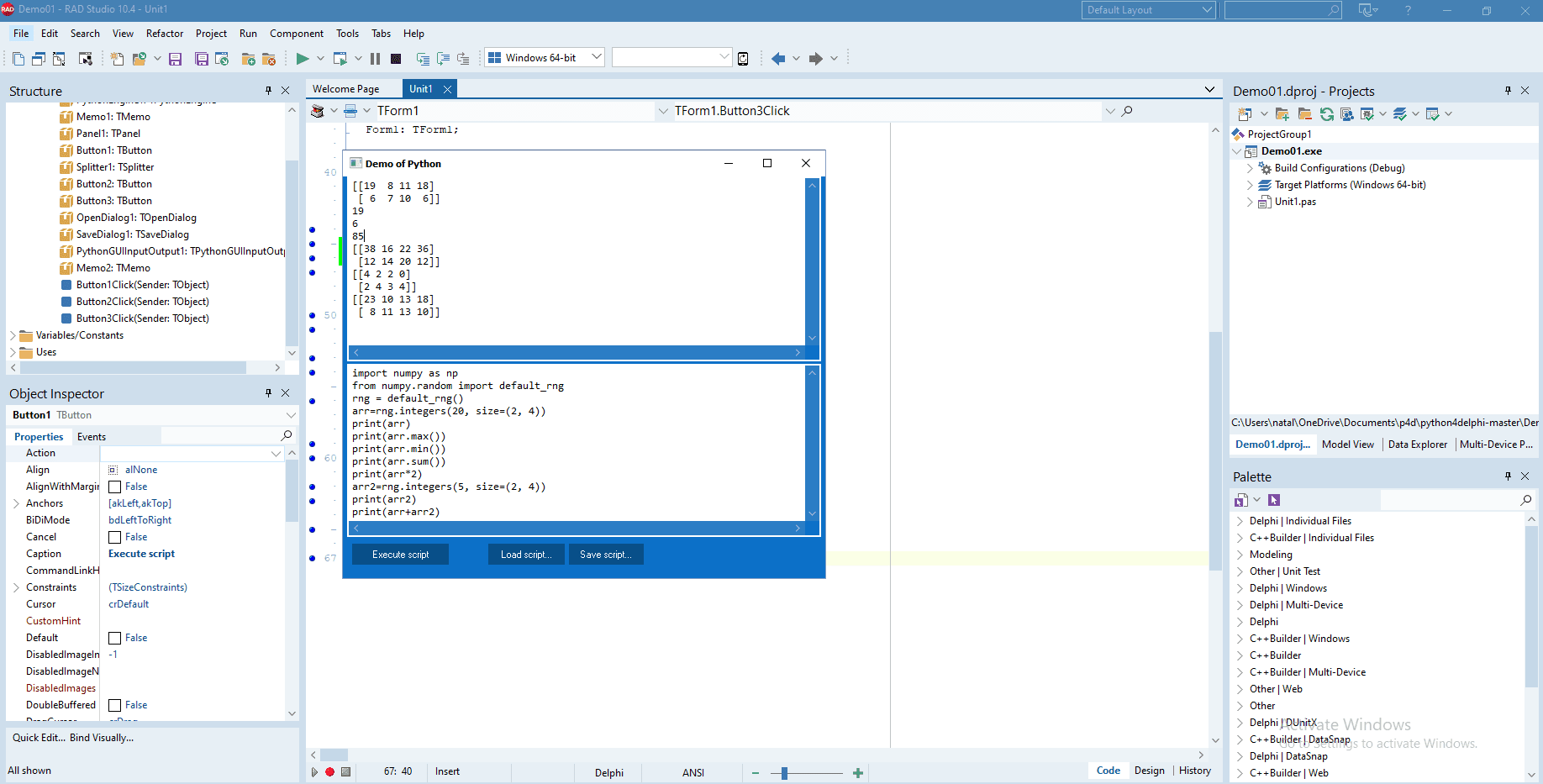Have an amazing solution built in RAD Studio? Let us know. Looking for discounts? Visit our Special Offers page!

# Learn To Build A Python GUI For Working With The Numpy Library In A Delphi Windows AppIf you need to perform complex transformations or mathematical calculations with matrices or arrays, then Python Numpy library is exactly what you need. You can easy run this library with Python4Delphi (P4D). Python4Delphi is a free tool with which you can work with Python scripts and objects in the Windows GUI. In this post, we will look at how to run Numpy library with P4D. Delphi itself offers a number of matrix multiplication features in TMatrix. If you have an existing Python application and you need matrix multiplication you could use the Numpy library or you could send the data to Delphi and do the multiplication there. Alternately, you could do all of your calculations in Numpy and then display it in the Delphi Windows GUI app. You can use Python4Delphi a number of different ways such as:
• Create a Windows GUI around you existing Python app.
• Enhance your speed sensitive Python apps with functions from Delphi for more speed.
Now you can build Python GUI apps for Windows using a lot of Python libraries with Delphi and C++Builder and Python4Delphi. Just open and run Demo1 project. Then paste the Python script into the text field, press Execute button and get the result. Go to GitHub to download Demo1. Numpy library allows you to create multidimensional arrays and matrices and work with their properties. It also contains various functions for processing arrays and matrices. Let’s look at some simple examples of working with Numpy. If you have trouble compiling the Python you may need to have Numpy 1.19.3 installed.

### Create a matrix and get some properties

This example shows how to create a 3-dimensional array and fill it with numbers from 0 to 29. Then, using the properties of this array, we can find out its shape, dimension, data type, number of elements.

### Basic operations with arrays

Let’s take a look at the simplest conversions you can perform on arrays. Using concatenate() function, you can combine the values of two arrays into one. With function sort() you can sort ascending the values in an array. Function reshape() allows you to change the dimension of the array.

### Mathematical operations with matrix

Function default_rng()  allows you to fill a matrix with random values. You can use integer or real numbers. In this example, we fill the matrix with integer values. Then we find the maximum and minimum element, the sum of all the elements in the matrix. It is also shown how you can multiply a matrix by a number and sum two matrices with the same dimension.And this is only a small part of what Numpy library allows you to do. Download Numpy and check how many possibilities it opens for your applications. Install Python4Delphi for building Python GUIs for Windows using Delphi easily.Reduce development time and get to market faster with RAD Studio, Delphi, or C++Builder.
Design. Code. Compile. Deploy.

••